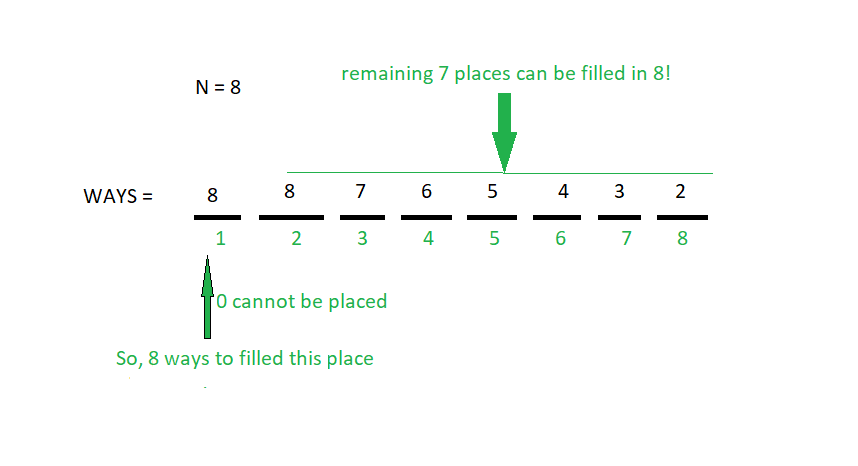# Count of N digit numbers possible which satisfy the given conditions

Given an integer N, the Task is to find the total number of N digit numbers possible such that:

1. All the digits of the numbers are from the range [0, N].
2. There are no leading 0s.
3. All the digits in a number are distinct.

Examples:

Input: N = 2
Output: 4
10, 12, 20 and 21 are the only possible 2 digit
numbers which satisfy the given conditions.

Input: N = 5
Output: 600

## Recommended: Please try your approach on {IDE} first, before moving on to the solution.

Approach: Given N number of digit and the first place can be filled in N ways [0 cannot be taken as the first digit and the allowed digits are from the range [1, N]]
Remaining (N – 1) places can be filled in N! ways
So, total count of number possible will be N * N!.

Take an example for better understanding. Say, N = 8First place can be filled with any digit from [1, 8] and the remaining 7 places can be filled in 8! ways i.e 8 * 7 * 6 * 5 * 4 * 3 * 2.
So, total ways = 8 * 8! = 8 * 8 * 7 * 6 * 5 * 4 * 3 * 2 = 322560

Below is the implementation of the above approach:

## C++

 `// C++ implementation of the approach ` `#include ` `using` `namespace` `std; ` ` `  `// Function to return the factorial of n ` `int` `fact(``int` `n) ` `{ ` `    ``int` `res = 1; ` `    ``for` `(``int` `i = 2; i <= n; i++) ` `        ``res = res * i; ` `    ``return` `res; ` `} ` ` `  `// Function to return the ` `// count of numbers possible ` `int` `Count_number(``int` `N) ` `{ ` `    ``return` `(N * fact(N)); ` `} ` ` `  `// Driver code ` `int` `main() ` `{ ` `    ``int` `N = 2; ` ` `  `    ``cout << Count_number(N); ` ` `  `    ``return` `0; ` `} `

## Java

 `// Java implementation of the approach ` `import` `java.io.*; ` ` `  `class` `GFG ` `{ ` ` `  `// Function to return the factorial of n ` `static` `int` `fact(``int` `n) ` `{ ` `    ``int` `res = ``1``; ` `    ``for` `(``int` `i = ``2``; i <= n; i++) ` `        ``res = res * i; ` `    ``return` `res; ` `} ` ` `  `// Function to return the ` `// count of numbers possible ` `static` `int` `Count_number(``int` `N) ` `{ ` `    ``return` `(N * fact(N)); ` `} ` ` `  `// Driver code ` `public` `static` `void` `main (String[] args)  ` `{ ` `    ``int` `N = ``2``; ` ` `  `    ``System.out.print(Count_number(N)); ` `} ` `} ` ` `  `// This code is contributed by anuj_67.. `

## Python3

 `# Python3 implementation of the approach ` ` `  `# Function to return the factorial of n ` `def` `fact(n): ` ` `  `    ``res ``=` `1` `    ``for` `i ``in` `range``(``2``, n ``+` `1``): ` `        ``res ``=` `res ``*` `i ` `    ``return` `res ` ` `  `# Function to return the ` `# count of numbers possible ` `def` `Count_number(N): ` `    ``return` `(N ``*` `fact(N)) ` ` `  `# Driver code ` `N ``=` `2` ` `  `print``(Count_number(N)) ` ` `  `# This code is contributed by Mohit Kumar `

## C#

 `// C# implementation of the approach ` `using` `System; ` ` `  `class` `GFG ` `{ ` ` `  `// Function to return the factorial of n ` `static` `int` `fact(``int` `n) ` `{ ` `    ``int` `res = 1; ` `    ``for` `(``int` `i = 2; i <= n; i++) ` `        ``res = res * i; ` `    ``return` `res; ` `} ` ` `  `// Function to return the ` `// count of numbers possible ` `static` `int` `Count_number(``int` `N) ` `{ ` `    ``return` `(N * fact(N)); ` `} ` ` `  `// Driver code ` `public` `static` `void` `Main ()  ` `{ ` `    ``int` `N = 2; ` ` `  `    ``Console.WriteLine(Count_number(N)); ` `} ` `} ` ` `  `// This code is contributed by anuj_67.. `

Output:

```4
```

My Personal Notes arrow_drop_upCheck out this Author's contributed articles.

If you like GeeksforGeeks and would like to contribute, you can also write an article using contribute.geeksforgeeks.org or mail your article to contribute@geeksforgeeks.org. See your article appearing on the GeeksforGeeks main page and help other Geeks.

Please Improve this article if you find anything incorrect by clicking on the "Improve Article" button below.

Improved By : mohit kumar 29, vt_m

Article Tags :
Practice Tags :

Be the First to upvote.

Please write to us at contribute@geeksforgeeks.org to report any issue with the above content.